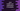# Bubble sort in Java with Examples## Bubble sort in Java :

Bubble sort repeatedly compares each elements in an array. We will traverse the array from the first element and check if it is greater than the second. If it is greater than the first, we will swap the first element with second. Then we will check the second element with third etc. This swapping will continue until no swap is needed.

In this tutorial, we will learn how to write bubble sort algorithm in Java. But before that, let’s try to understand with a simple example : ( We will sort the array (5,4,3,2) )

First pass : Scan from first to third element :

``````(5,4,3,2) -> (4,5,3,2) : compare 1st,2nd elements : 5>4 , they are swapped
(4,5,3,2) -> (4,3,5,2) : compare 2nd,3rd elements : 5>3 , they are swapped
(4,3,5,2) -> (4,3,2,5) : compare 3rd,4th elements : 5>2 , they are swapped
``````

Second Pass : Since the largest element is moved to 4th , scan from first to Second element :

``````(4,3,2,5) -> (3,4,2,5) : compare 1st,2nd elements : 4>3 , they are swapped
(3,4,2,5) -> (3,2,4,5) : compare 2nd,3rd elements : 4>2 , they are swapped
``````

Third Pass : Since the largest element is moved to 3th , we will check only first two elements :

``````(3,2,4,5) -> (2,3,4,5) : compare 1st,2nd elements : 3>2 , they are swapped
``````

So, you can see that we have used three ‘pass’ and sort the full array. You can think about this ‘pass’ as the loop iteration . First ‘pass’ means first time loop is iterating, second ‘pass’ means second time loop is iterating etc.

### Time complexity :

Best case : If the list is already sorted, it is the best case. In this case, the complexity is O(n).

Worst Case : If the list is inverted, the complexity is O(n2).

### Java program of Bubble sort :

• Following Java program explains the bubble sort .

• We are using one ‘while’ loop that will run continuously till the whole list is sorted.

• One flag ‘isCompleted’ is used to detect if all elements are sorted or not.

• ‘printArray(int[] arr)’ is used to print elements of an array.

• We call this method to print the array before and after the sorted is done. Let’s take a look into the program

``````public class Main {

/**
* Bubble sort one array
*
* @param arr : array to sort
*/
public static void bubbleSort(int[] arr) {
int length = arr.length;
boolean isCompleted = false;

while (!isCompleted) {
isCompleted = true;
for (int i = 0; i < length - 1; i++) { if (arr[i] > arr[i + 1]) {
int temp = arr[i + 1];
arr[i + 1] = arr[i];
arr[i] = temp;
isCompleted = false;
}
}
}
}

/**
* Print one array
*
* @param arr : array to print
*/
public static void printArray(int[] arr) {
for (int i = 0; i < arr.length; i++) {
System.out.print(arr[i]);
if (i != arr.length - 1) {
System.out.print(",");
}
}
System.out.println();
}

/**
* Helper method to sort an array
*
* @param arr : Array to sort
*/
public static void bubbleSortArray(int[] arr) {
System.out.println("Array before sorting :");
printArray(arr);
bubbleSort(arr);
System.out.println("Array after sorting :");
printArray(arr);
}

public static void main(String[] args) {
int arr[] = {5, 4, 3, 2, 1};
bubbleSortArray(arr);

int arr1[] = {1, 4, 3, 2, 5};
bubbleSortArray(arr1);

int arr2[] = {1, 2, 5, 4, 3, 7, 6, 14, 9};
bubbleSortArray(arr2);
}
}
``````

#### Output :

``````Array before sorting :
5,4,3,2,1
Array after sorting :
1,2,3,4,5
Array before sorting :
1,4,3,2,5
Array after sorting :
1,2,3,4,5
Array before sorting :
1,2,5,4,3,7,6,14,9
Array after sorting :
1,2,3,4,5,6,7,9,14
``````